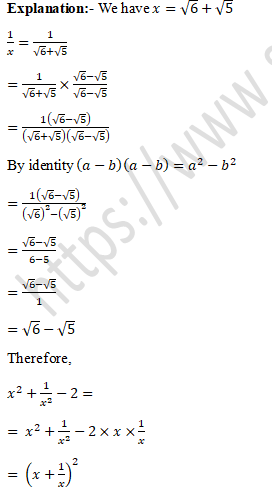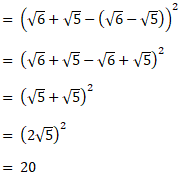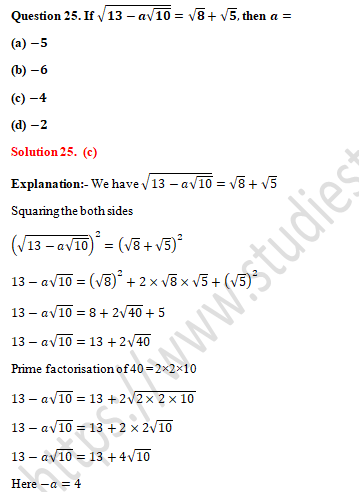# RD Sharma Solutions Class 9 Chapter 3 Rationalisation

Read RD Sharma Solutions Class 9 Chapter 3 Rationalisation below, students should study RD Sharma class 9 Mathematics available on Studiestoday.com with solved questions and answers. These chapter wise answers for class 9 Mathematics have been prepared by teacher of Grade 9. These RD Sharma class 9 Solutions have been designed as per the latest NCERT syllabus for class 9 and if practiced thoroughly can help you to score good marks in standard 9 Mathematics class tests and examinations

Exercise 3.1
Question 1. Simplify each of the following:Solution 1.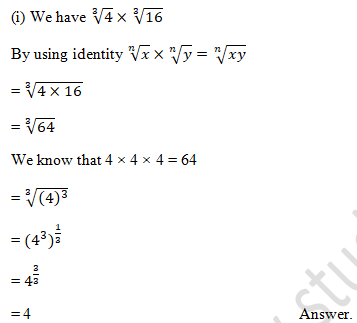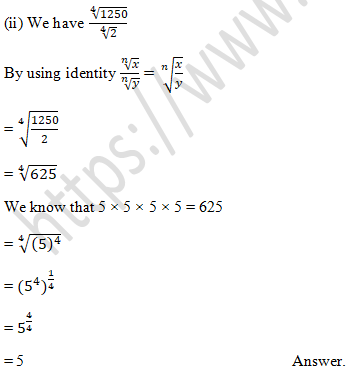Question 2. Simplify the following expressions:
(i) (4+√7) (3+√2)
(ii) (3+√3) (5-√2)
(iii) (√5-2) (√3-√5)
Solution 2.
(i) We have (4+√7) (3+√2)
By using identity √x×√y=√xy
= 4(3+√2) + √7(3+√2)
= 4×3 + 4×√2 + 3×√7 + √2×√7
= 12 + 4√2 + 3√7 + √14                                                  Answer.

(ii) We have (3+√3) (5-√2)
By using identity √x×√y=√xy
= 3(5-√2) + √3(5-√2)
= 3×5 - 3×(√2) + √3×5 + √3×(-√2)
= 15 - 3√2 + 5√5 - √6                                                  Answer.

(iii) We have (√5-2) (√3-√5)
By using identity √x×√y=√xy
= √5(√3-√5) - 2(√3-√5)
= √5×√3 – √5 × √5 + 2×√3 + 2 ×√5
= √15 - √25 + 2√3 - 2√5
= √15 - 5 - 2√3 - 2√5                                                 Answer.

Question 3. Simplify the following expressions:
(i) (11+√11) (11-√11)
(ii) (5+√7) (5-√7)
(iii) (√8-√2) (√8+√2)
(iv) (3+√3) (3-√3)
(v) (√5-√2) (√5+√2)
Solution 3.
(i) We have (11+√11)(11-√11)
By using identity (a+b)  (a-b)=a2-b2
= (11)2-(√11)2
= 121-11

(ii) We have (5+√7) (5-√7)
By using identity (a+b)  (a-b)=a2-b2
= (5)2-(√7)2
= 25-7

(iii) We have (√8-√2) (√8+√2)
By using identity (a+b)  (a-b)=a2-b2
= (√8)2-(√2)2
= 8-2

(iv) We have (3+√3) (3-√3)
By using identity (a+b)  (a-b)=a2-b2
= (3)2-(√3)2
= 9-3

(v) We have (√5-√2) (√5+√2)
By using identity (a+b)  (a-b)=a2-b2
= (√5)2-(√2)2
= 5-2

Question 4. Simplify the following expressions:
(i) (√3+√7)2
(ii) (√5-√3)2
(iii) (2√5+3√2)2
Solution 4.
(i) We have (√3+√7)2
By using identity (a+b)2= a2+2ab+b2 and √a×√b=√ab
=(√3)2+2√3 √7+(√7)2
=3+2√21+7

(ii) We have (√5+√3)2
By using identity (a-b)2= a2-2ab+b2 and √a×√b=√ab
=(√5)2-2√5 √3+(√3)2
=5-2√15+3

(iii) We have (2√5+3√2)2
By using identity (a+b)2= a2+2ab+b2 and √a×√b=√ab
=(2√5)2+2×2√5×3√2+(3√2)2
=22×(√5)2+2×2√5×3√2+32×(√2)2
=4×5+12√10+9×2
=20+12√10+18

Exercise 3.2
Question 1. Rationalise the denominator of each of the following:-
(i) 3/√5
(ii) 2/(2√5)
(iii) 1/√12
(iv) √2/√5
(v) (√3+1)/√2
(vi) (√2+√5)/√3
(vii) (3√2)/√5
Solution 1.
(i) We have 3/√5
Here  3/√5 as an equivalent expression in which the denominator is a rational number. We know that √5×√5=5 is a rational. We also know that if we multiplying 3/√5 by √5/√5 will gives us an equivalent expression, since √5/√5 = 1.
=3/√5×√5/√5
= (3 × √5)/(√5  × √5)

(ii) We have 3/(2√5)
Here  3/√5 as an equivalent expression in which the denominator is a rational number. We know that √5×√5=5 is a rational. We also know that if we multiplying 3/(2√5) by √5/√5 will gives us an equivalent expression, since √5/√5 = 1.
=3/(2√5)×√5/√5
= (3 × √5)/(2×√5  × √5)
= (3√5)/(2×5)

(iii) We have 1/√12
Here  1/√12 as an equivalent expression in which the denominator is a rational number. We know that √12×√12=12 is a rational. We also know that if we multiplying 1/√12 by √12/√12 will gives us an equivalent expression, since √12/√12 = 1.
=1/√12×√12/√12
= (1 × √12)/(√12  × √12)
= (1√12)/12
= √12/12
By Prime factorisation of 12 we get = 2×2×3
= √(2×2×3)/12

(iv) We have √2/√5
Here  √2/√5 as an equivalent expression in which the denominator is a rational number. We know that √5×√5=5 is a rational. We also know that if we multiplying √2/√5 by √5/√5 will gives us an equivalent expression, since √5/√5 = 1.
=√2/√5×√5/√5
= (√2  × √5)/(√5  × √5)

(v) We have (√3+1)/√2
Here  (√3+1)/√2 as an equivalent expression in which the denominator is a rational number. We know that √2×√2=2 is a rational. We also know that if we multiplying (√3+1)/√2 by √2/√2 will gives us an equivalent expression, since √2/√2 = 1.
=(√3+1)/√2×√2/√2
= ((√3+1)  × √2)/(√2  × √2)
= ((√2×√3)+(√2×1))/2

(vi) We have (√2+√5)/√3
Here  (√2+√5)/√3 as an equivalent expression in which the denominator is a rational number. We know that √3×√3=3 is a rational. We also know that if we multiplying (√2+√5)/√3 by √3/√3 will gives us an equivalent expression, since √3/√3 = 1.
=(√2+√5)/√3×√3/√3
= ((√2+√5)  × √3)/(√3  × √3)
= ((√2×√3)+(√5×√3))/2

(vii) We have √2/√5
Here  √2/√5 as an equivalent expression in which the denominator is a rational number. We know that √5×√5=5 is a rational. We also know that if we multiplying √2/√5 by √5/√5 will gives us an equivalent expression, since √5/√5 = 1.
=(3√2)/√5×√5/√5
= (3√2  × √5)/(√5  × √5)

Question 2.  Find the value to three places of decimals of each of the following. It is given that √2=1.414,√3=1.732,√5=2.236 and √10=3.162.
(i) 2/√3
(ii) 3/√10
(iii) (√5+1)/√2
(iv) (√10+√15)/√2
(v) (2+√3)/3
(vi) (√2-1)/√5
Solution 2.
(i) We have   2/√3
Rationalising of denominator is √3. We know that √3×√3=3 is a rational. We also know that if we multiplying 2/√3 by √3/√3 will gives us an equivalent expression, since √3/√3 = 1.
=2/√3×√3/√3
= (2 × √3)/(√3  × √3)
= (2√3)/3
It is given that the value of is√3=1.732.
= (2 ×1.732)/3
= 3.464/3

(ii) We have   3/√10
Rationalising of denominator is √10. We know that √10×√10=10 is a rational. We also know that if we multiplying 3/√10 by √10/√10 will gives us an equivalent expression, since √10/√10 = 1.
=3/√10×√10/√10
= (3 × √10)/(√10  × √10)
= (3√10)/10
It is given that the value of is√10=3.162.
= (3 × 3.162)/10
= 9.486/10

(iii) We have   (√5+1)/√2
Rationalising of denominator is √2. We know that √2×√2=2 is a rational. We also know that if we multiplying (√5+1)/√2 by √2/√2 will gives us an equivalent expression, since √2/√2 = 1.
=(√5+1)/√2×√2/√2
= (√2 (√5+1))/(√2  × √2)
= ((√2×√5)+(1×√2))/2
= (√10+√2)/2
It is given that the value of is √10=3.162 and √2=1.414
= (3.162 + 1.414)/2
= 4.576/2

(iv) We have   (√10+√15)/√2
Rationalising of denominator is √2. We know that √2×√2=2 is a rational. We also know that if we multiplying (√10+√15)/√2 by √2/√2 will gives us an equivalent expression, since √2/√2 = 1.
=(√10+√15)/√2×√2/√2
= (√2 (√10+√15))/(√2  × √2)
= ((√2×√10)+(√2×√15))/2
= (√20+√30)/2
= (√(2×10)+√(3×10))/2
= (√2  × √10  + √3  × √10)/2
It is given that the value of is √10=3.162, √2=1.414 and √3=1.732
= ((1.414 ×3.162)+(1.732×3.612)  )/2
= (4.471 +5.476)/2
= 9.947/2

(v) We have (2+√3)/3
It is given that the value of is √3=1.732
= (2 + 1.732)/3
= 3.732/3

(vi) We have (√2-1)/√5
Rationalising of denominator is √5. We know that √5×√5=5 is a rational. We also know that if we multiplying (√2  + 1)/√5 by √5/√5 will gives us an equivalent expression, since √5/√5 = 1.
=(√2  + 1)/√5×√5/√5
= (√5 (√2-1))/(√5  × √5)
= ((√5  × √2)-(√5  × 1))/5
= (√10-√5)/5
It is given that the value of is √10=3.162, and √5=2.236
= (3.162-2.236)/5
= 0.926/5

Question 3.  Express each one of the following with rational denominator:
(i) 1/(3+√2)          (vi) (√3+1)/(2√2-√3)
(ii) 1/(√6-√5)       (vii) (6-4√2)/(6+4√2)
(iii) 16/(√41-5)     (viii) (3√2+1)/(2√5-3)
(iv) 30/(5√3-3√5) (ix) b2/(√(a2+b2 )+a)
(v) 1/(2√5-√3)
Solution 3.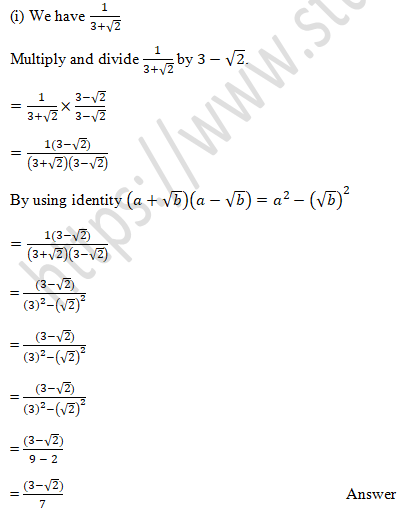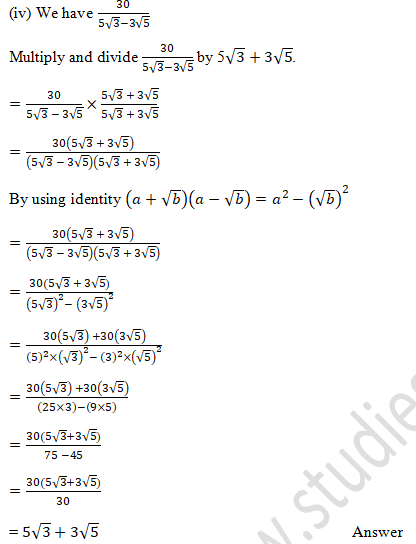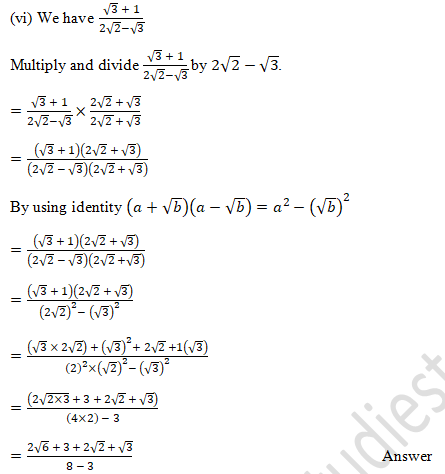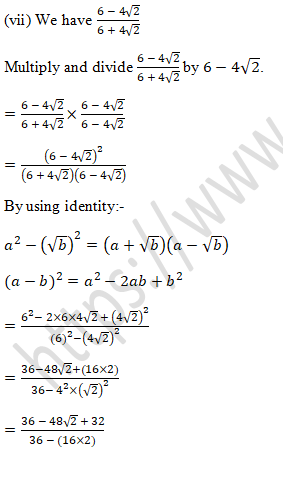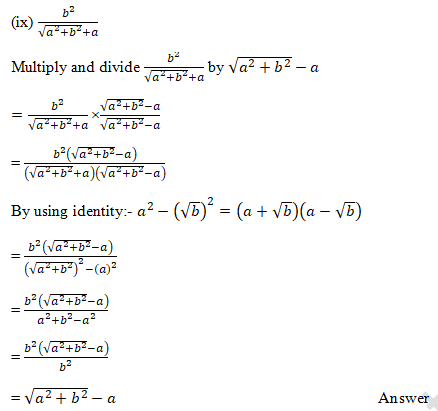Question 4.  Rationalize the denominator and simplify:Solution 4.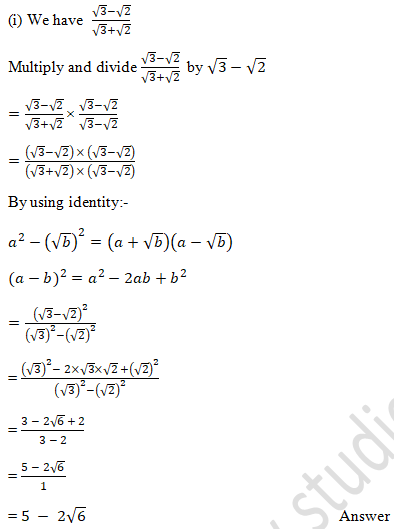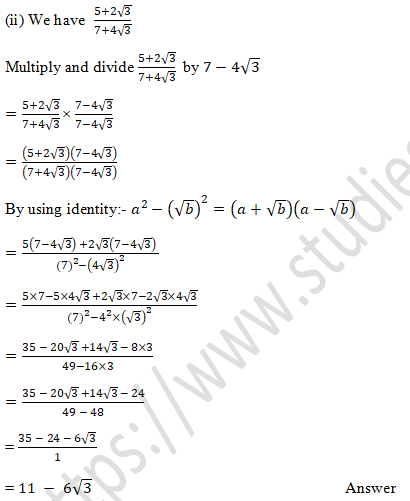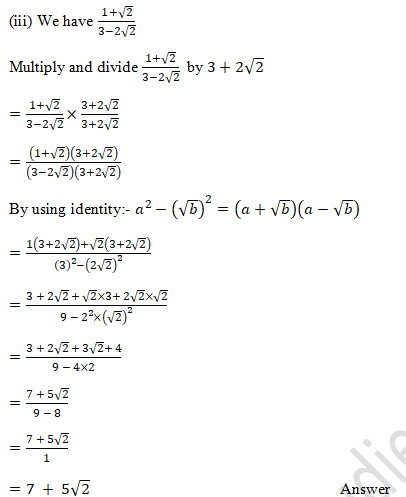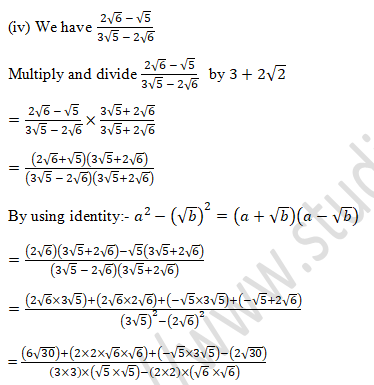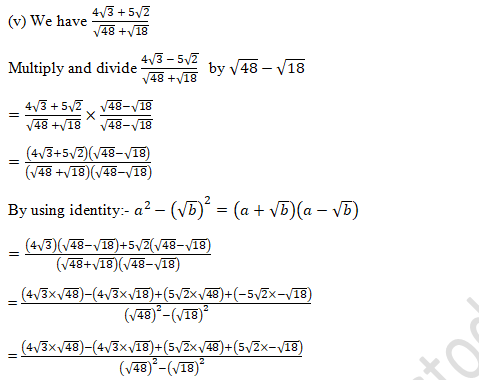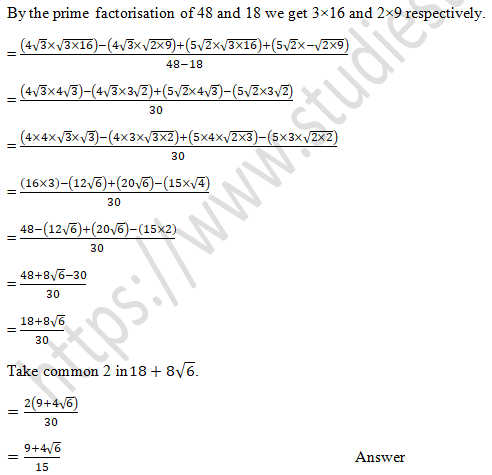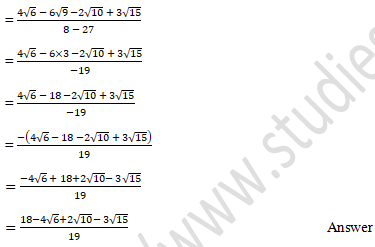Question 5. Simplify: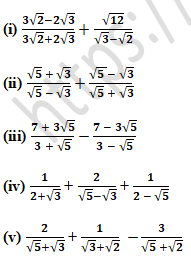Solution 5.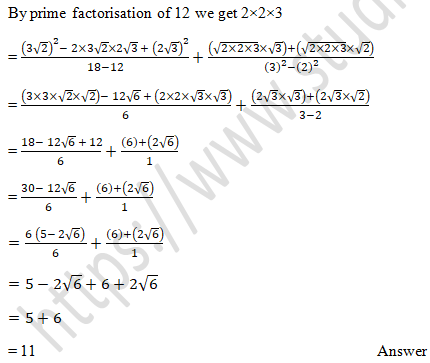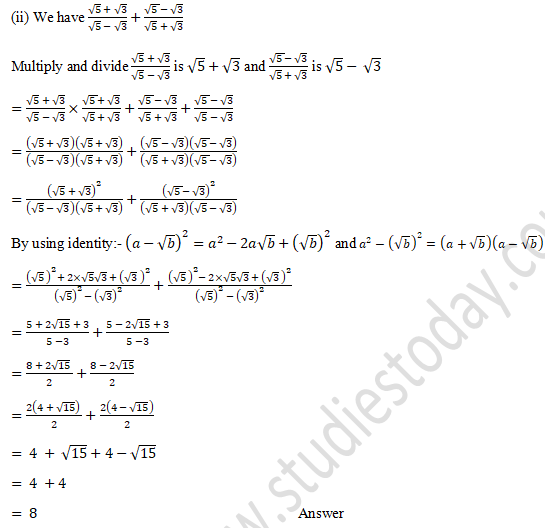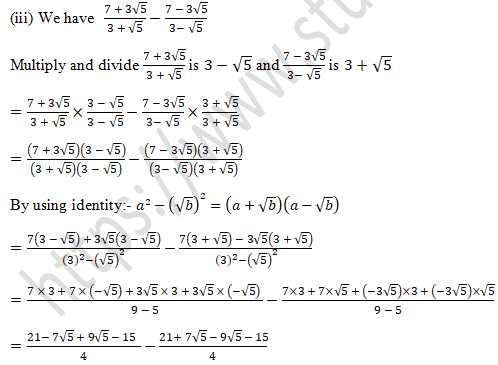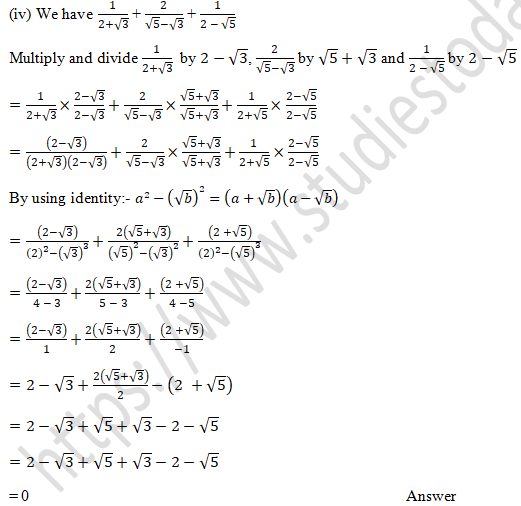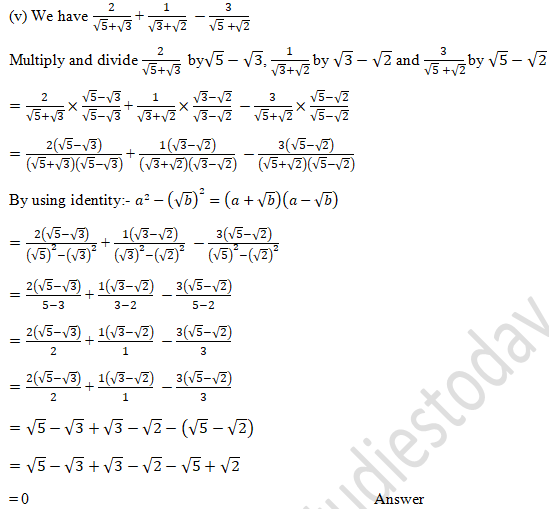Question 6. In each of the following determine rational numbers a and b:Solution 6.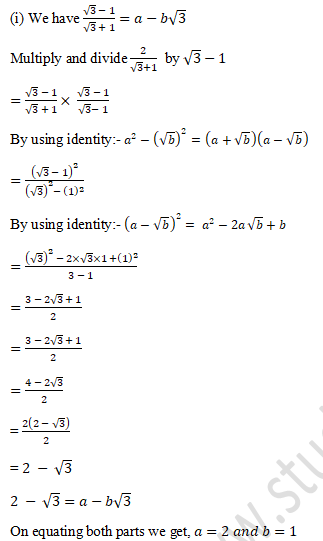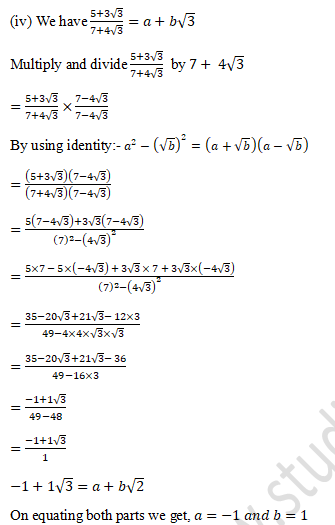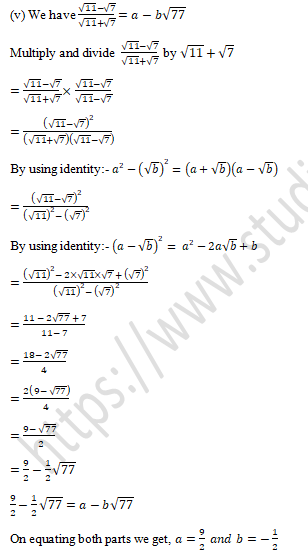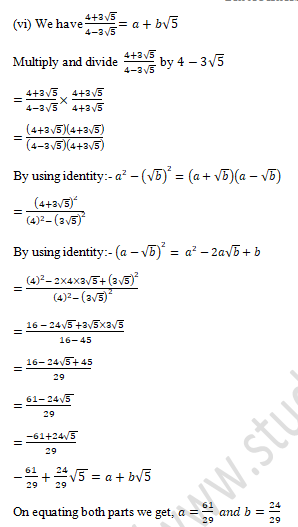Question 7. If x = 2 + √3, find the value of x3+1/x3 .
Solution 7.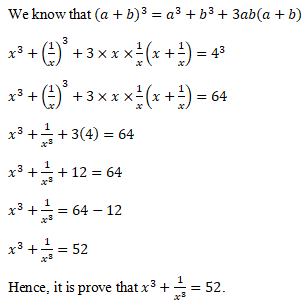Question 8. If x = 3 + √8, find the value of x2+1/x2
Solution 8.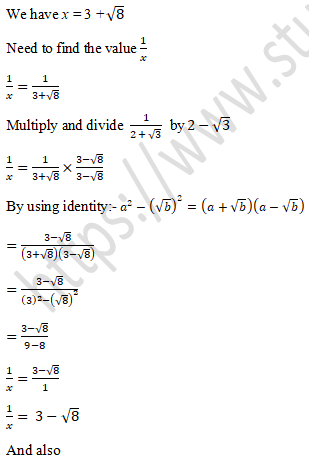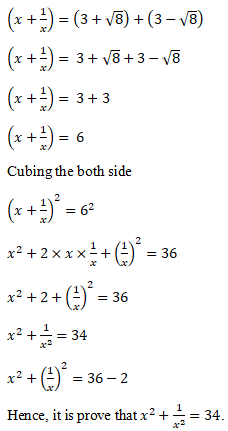Question 9.  Find the value of6/(√5-√3) it being given that √3=1.732 and √5=2.236.
Solution 9.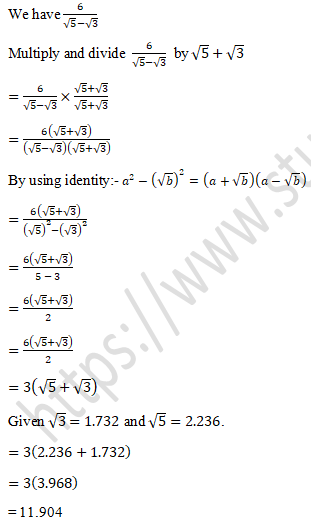Question 10. Find the value of to three places if decimals, it being given that √2=1.4142,√3=1.732,√5=2.2360,√6=2.4495 and √10=3.162.
(i) (3-√5)/(3+2√5)
(ii) (1+√2)/(3-2√2)
Solution 10.
We have (3-√5)/(3+2√5)
Multiply and divide  (3-√5)/(3+2√5)  by 3-2√5
(3-√5)/(3+2√5)×(3-2√5)/(3-2√5)
(3-√5)(3-2√5)/(3+2√5)(3-2√5)
By using identity:- a2-(√b)2=(a+√b)(a-√b)
=(3-√5)(3-2√5)/((3)2-(2√5)2
=((3)(3-2√5)-√5 (3-2√5))/(9-2×2×√5×√5)
=((3×3)+3×(-2√5)-√5×3-√5×(-2√5))/(9-4×5)
=(9 - 6√5  - 3√5  +10)/(9-20)
=(19 - 9√5  )/(-11)
=(19 - 9×2.2360 )/(-11)
=(19 - 20.124 )/(-11)
=(- 1.1240 )/(-11)
=(1.1240 )/11

(ii) We have (1+√2)/(3-2√2)
Multiply and divide  (1+√2)/(3-2√2)  by 3-2√2
(1+√2)/(3-2√2)×(3+2√2)/(3+2√2)
(1-√2)(3+2√2)/(3-2√5)(3+2√2)
By using identity:- a2-(√b)2=(a+√b)(a-√b)
=(1-√2)(3-2√2)/((3)2-(2√2)2
=((1)(3+2√2)-√2 (3-2√2))/(9-2×2×√2×√2)
=((1×3)+1×(2√2)-√2×3-√2×(-2√2))/(9-4×2)
=(3+2√2+3√2-√2×(-2√2))/(9-8)
=(3+2√2+3√2+4)/1
=(7+5√2)/1
=7+5√2
=7+5×1.4142
=7+7.0710

Question 11.  If x = (√3+1)/2, find the value of 4x3+2x2-8x+7.
Solution 11.Question 1. Write the value of (2+√3)(2-√3)
Solution 1.
We have (2+√3)(2-√3).
By identity (a+b)(a-b)=a2-b2
=(2)2-(√3)2
=4-3
=1                                                                        Ans.

Question 2. Write the reciprocal of 5+√2.
Solution 2.
We have 5+√2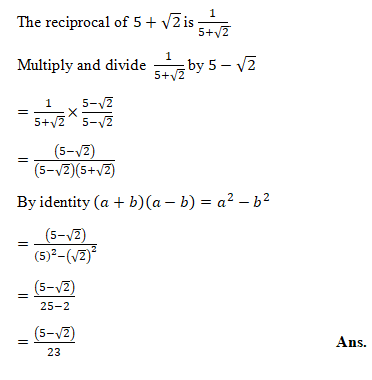Question 3. Write the rationalisation factor of 7-3√5.
Solution 3.
We know that the rationalised factor of (x+√y) is (x-√y)
The rationalised factor of (7-3√5) is (7+3√5)

Question 4. If (√3-1)/(√3+1)=x+y√3, find the values of x and y.
Solution 4.
We have (√3-1)/(√3+1)=x+y√3

Multiply and divide  (√3-1)/(√3+1) by √3-1.

=(√3-1)/(√3+1)×(√3-1)/(√3-1)

=(√3-1)2/(√3+1)(√3-1)

By identity (a+b)(a-b)=a2-b2  and (a-b)2=a2-2ab+b2

=((√3)2-2×√3×1 + (1)2)/((√3)2-(1)2

=(3-2√3  + 1)/(3-1)

=(4 - 2√3  )/2

=(2(2-√3)  )/2

2-√3 =x+y√3

Hence, the value of  x=2 and y=-1

Question 5. If x=√2-1, then write the value of 1/x.
Solution 5.
We have x=√2-1.
1/x=1/(√2-1)
For Rationalise 1/(√2-1) we have to Multiply and divide  1/(√2-1)  by √2+1.
=1/(√2-1)×(√2+1)/(√2+1)
=1(√2+1)/(√2-1)(√2+1)
By identity (a+b)(a-b)=a2-b2
=((√2+1))/((√2)2-(1)2
=((√2+1))/(2-1)
=((√2+1))/1
=(√2+1)                                                                       Ans.

Question 6.  If a=(√2+1), then find the value of a-1/a.
Solution 6.
It is given that a=(√2+1)
1/a=1/((√2+1) )
For Rationalise 1/(√2+1) we have to Multiply and divide  1/(√2+1)  by √2-1.
=1/(√2+1)×(√2-1)/(√2-1)
=((√2-1))/(√2+1)(√2-1)
=((√2-1))/((√2)2-(1)2
=((√2-1))/(2-1)
=((√2-1))/1
=√2-1
Therefore,
a-1/a=(√2+1)-√2-1
a-1/a=√2+1-√2+1
a-1/a=1+1
a-1/a=2                                                             Ans.

Question 7.  If a=(2+√2), find the value of x-1/x.
Solution 7.
It is given that x=(2+√2)
1/x=1/((2+√2) )
For Rationalise 1/(2+√2) we have to Multiply and divide  1/(2+√2)  by 2-√2.
=1/(2+√2)×(2-√2)/(2-√2)
=((2-√2))/(2+√2)(2-√2)
=((2-√2))/((2)2-(√2)2
=((2-√2))/(4-2)
=((2-√2))/2
=2-√2
Therefore,
x+1/x=(2+√2)+(2-√2)
x-1/x=2+√2+2-√2
x-1/x=2+2
x-1/x=4                                                                       Ans.

Question 8.  Write the rationalisation factor of √5-2.

Solution 8.
We know that the rationalised factor of (x+√y) is (x-√y)

The rationalised factor of (√5-2) is (√5+2)

Question 9. Simplify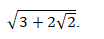Solution 9.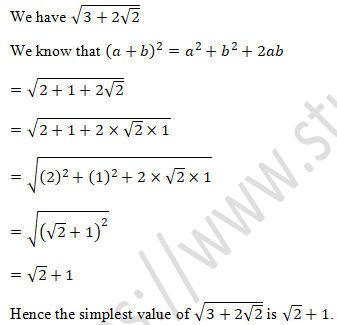Question 10. Simplify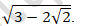Solution 10.Question 11. If x=3+2√2, then find the value of √x-1/√x.
Solution 11.
It is given that x=3+2√2
We need to find Value of √x-1/√x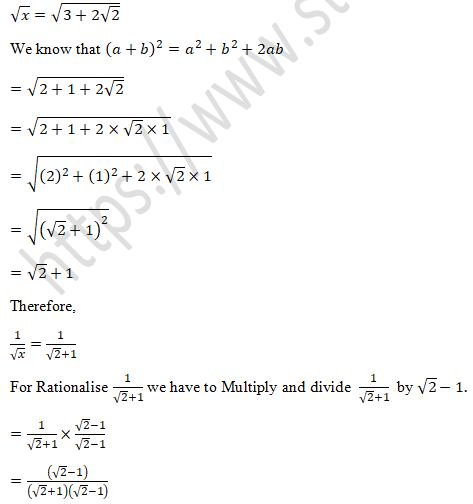Multiple Choice Questions (MCQs).....................................

Question 1.  √10×√15  is equal to

(a) 5√6

(b) 6√5

(c) √30

(d) √25

Solution 1.  (a)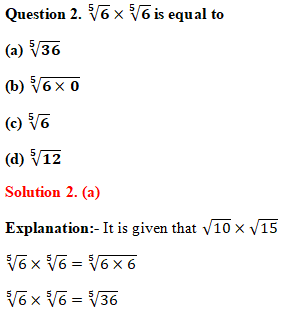Question 3.  The rationalisation factor of √3 is
(a) -√3
(b) 1/√3
(c) 2√3
(d) -2√3
Solution 3.  (b)
Explanation:- We know that the rationalised factor of √x is 1/√x
The rationalised factor of (√3) is 1/√3.

Question 4.  The rationalisation factor of 2+√3is.
(a) 2-√3
(b) 2+√3
(c) √2-3
(d) √3-2
Solution 4.  (a)
Explanation:- We know that the rationalised factor of x+√y is (x-√y)
The rationalised factor of (2+√3) is (2-√3).

Question 5.  If x=√5+2, than x-1/x equals.
(a) 2√5
(b) 4
(c) 2
(d) √5
Solution 5. (b)Question 6.  If √(3-1)/(√3+1)=a-b√3, then
(a) a = 2, b = 1
(b) a = 2, b = -1
(c) a = -2, b = 1
(d) a = b = 1
Solution 6.   (a)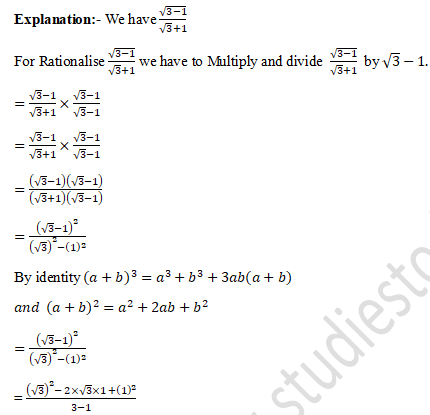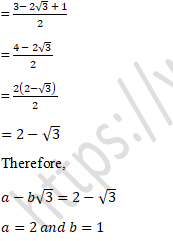Question 8. The simplest rationalising factor of √3+√5 is.
(a) √3-5
(b) 3-√5
(c) √3-√5
(d) √3+√5
Solution 8.  (c)
Explanation:- We know that the rationalised factor of √x+√y is (√x-√y)
The rationalised factor of (√3+√5) is (√3-√5).

Question 9.  The simplest rationalising factor of 2√5-√3 is
(a) 2√5+3
(b) 2√5+√3
(c) √5+√3
(d) √5-√3
Solution 9.  (b)
Explanation:- We know that the rationalised factor of a√x-√y is (a√x+√y)
The rationalised factor of (2√5-√3) is (2√5+√3).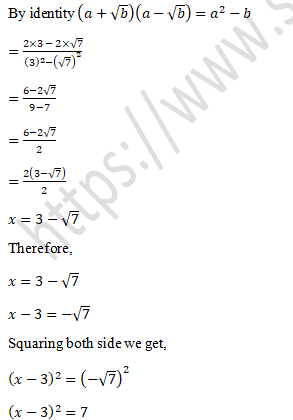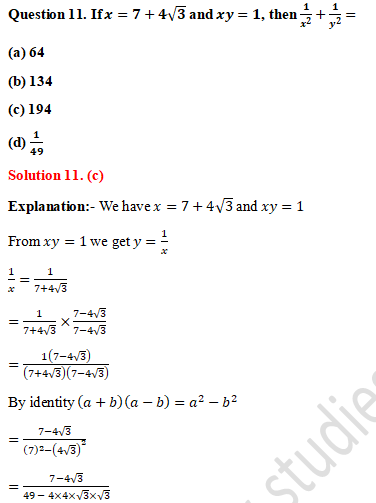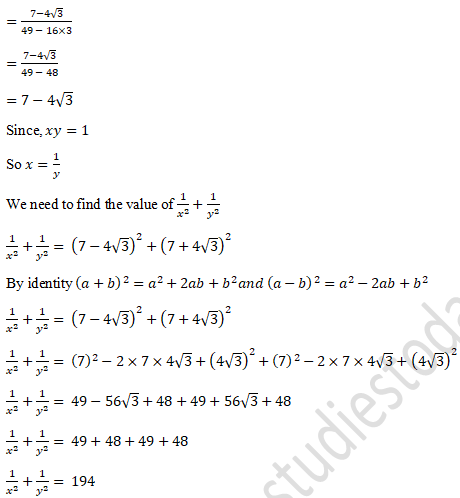Question 12.  If x+√15=4, then x+1/x
(a) 2
(b) 4
(c) 8
(d) 1
Solution 12.  (c)Question 13. If x=(√5+√3)/(√5-√3) and y=(√5-√3)/(√5+√3), then x+y+xy=
(a) 9
(b) 5
(c) 17
(d) 7
Solution 13.  (a)Question 14. If x=(√3-√2)/(√3+√2) and y=(√3+√2)/(√3-√2), then x2+xy+y2=
(a) 101
(b) 99
(c) 98
(d) 102
Solution 14.  (b)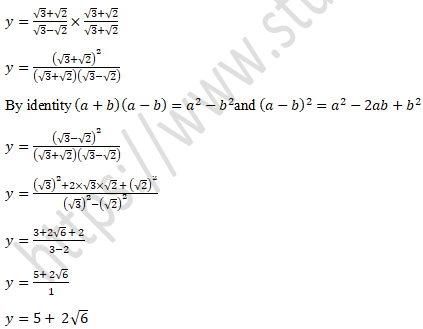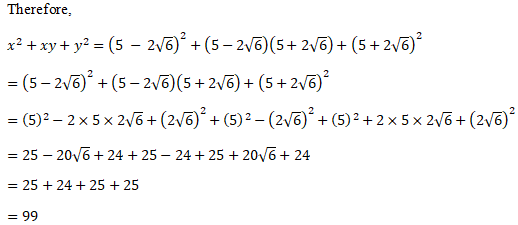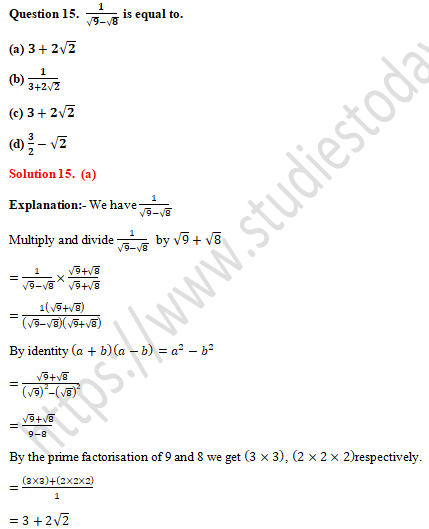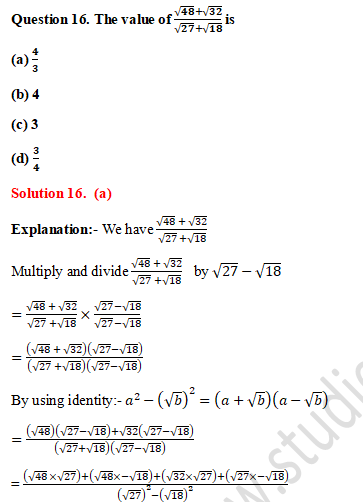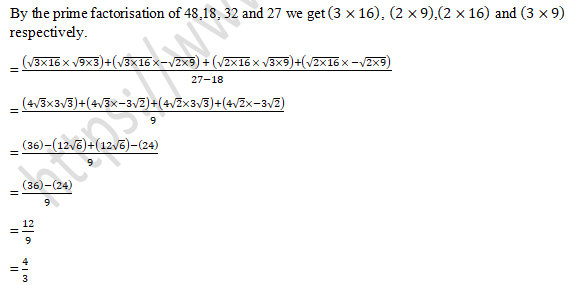Question 17.  If (5-√13)/(2+√3)=x+y√3, then
(a) x=13,y=-7
(b) x=-13,y=7
(c) x=-13,y=-7
(d) x=13,y=7
Solution 17.  (a)
Explanation:- We have  (5-√13)/(2+√3)
We need to find the value of x and y
Multiply and divide (5-√13)/(2+√3)  by 2-√3
=(5-√13)/(2+√3)×(2-√3)/(2-√3)
=(5-√3)(2-√3)/(2+√3)(2-√3)
By identity (a+b)(a-b)=a2-b2
=(5-√3)(2-√3)/((2)2-(√3)2 )
=(5-√3)(2-√3)/(4-3)
=(5(2-√3)-√13 (2-√3))/1
=5×2-5×√3-2√3-√3×√3
=10-5√3-2√3-3
=13-7√3
Therefore,
x+y√3=13-7√3
x=13 and y=-7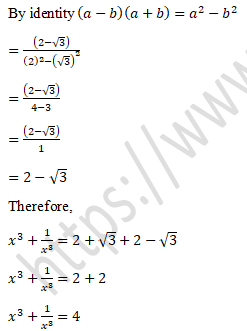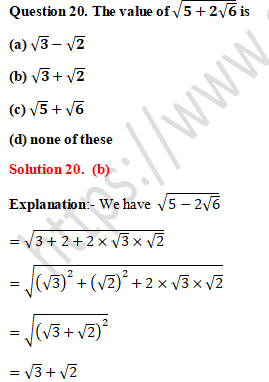Question 22.  If √2=1.414, then √6-√3 upto three places of decimal is
(a) 0.235
(b) 0.707
(c) 1.414
(d) 0.471
Solution 22.  (b)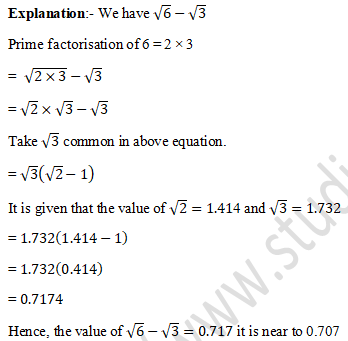Question 23.  The positive square root of 7+√48 is
(a) 7+2√3
(b) 7+√3
(c) 2+√3
(d) 3+√2
Solution 23.  (c)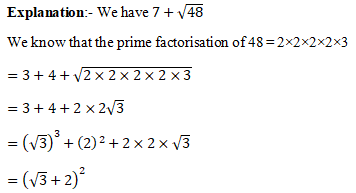Question 24. If x=√6+√5, then x2+1/x2 -2=
(a) 2√6
(b) 2√5
(c) 24
(d) 20
Solution 24.  (d)